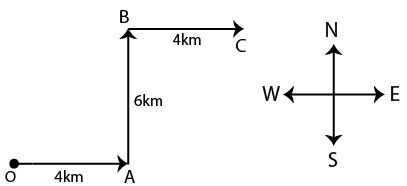Question 186

# Sonia started from her house and travelled 4 km towards east. Then she turned left and travelled 6 km. Then she turned right and travelled 4 km. Now at what distance is she from starting point ?

Solution

The path of Sonia can be traced asthe distance between her start and finish points will be equal to OC, which by using pythagoras theorem will be:
$$\sqrt{8^{2}+6^{2}}=10$$. therefore the correct answer is option D.

• Free SSC Study Material - 18000 Questions
• 230+ SSC previous papers with solutions PDF